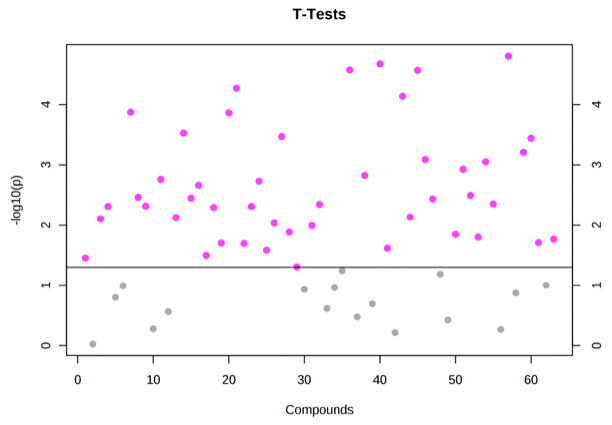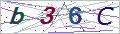Services

Online Inquiry

# T-test Analysis Service

A t-test is a statistical hypothesis test in which the test statistic obeys a Student's t-distribution under the null hypothesis. It can be used to test whether two datasets are significantly different from each other. A t-test is mostly applied when the values of a scaling term in the test statistic are known and follow a random distribution pattern. When the scaling term is unknown and is replaced by an estimate according to the data, the test statistics (under some conditions) follow a Student's t distribution.Two-sample t-tests for a difference in mean include independent samples or unpaired samples. The independent samples t-test is applied when two separate sets of independent and identically distributed samples are available, one from each of the two populations being compared. Paired samples t-test is typically composed of a sample of matched pairs of similar units, or one group of units that has been tested twice (a "repeated measures" t-test). Paired t-tests are a type of blocking, and is more accurate than unpaired tests when the paired units are similar with respect to "noise factors". In a different context, paired t-tests can be applied to reduce the influence of confounding factors in an observational study.

Below shows the commonly used t-tests:

• A one-sample location test of whether the mean of a given population has a value specified in a null hypothesis.
• A two-sample location tests of the null hypothesis such that the means of two populations are equal. All such tests are often called Student's t-tests, though strictly speaking this name should only be applied if the variances of these two populations are also supposed to be equal; the form of the test used when this assumption is dropped is occasionally called Welch's t-test. These tests are usually referred to as "unpaired" or "independent samples" t-tests, as they are typically used when the statistical units underlying the two samples to be compared are non-overlapping.
• A test of the null hypothesis that the difference between two responses measured on the same statistical unit has a mean value of zero. For instance, suppose we measure the size of a cancer patient's tumor before and after a treatment. If this treatment is effective, we suppose the tumor size for many of the patients to be smaller following the treatment. This is usually referred to as the "paired" or "repeated measures" t-test.
• A test of whether the slope of a regression line is significantly different from zero.

How to place an order:* For Research Use Only. Not for use in diagnostic procedures.
Our customer service representatives are available 24 hours a day, 7 days a week. Inquiry

Online Inquiry

Please submit a detailed description of your project. We will provide you with a customized project plan to meet your research requests. You can also send emails directly to for inquiries.

* Email
Phone
* Service & Products of Interest
Services Required and Project Description
* Verification Code